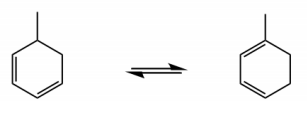Start typing, then use the up and down arrows to select an option from the list.# Organic Chemistry

Learn the toughest concepts covered in Organic Chemistry with step-by-step video tutorials and practice problems by world-class tutors.

16. Conjugated Systems

# Sigmatropic Rearrangement

Here we will discuss an intramolecular pericyclic reaction in which 0 pi bonds are destroyed after a cyclic mechanism.

1

#### concept

Definition of Sigmatropic Shifts3m
Play a video:
in this video, we're gonna discuss a type of Paris cyclic reaction called a Sigma Tropic Shift. So what is a Sigma Tropic shift? Well, it's an intra molecular Perry cyclic reaction in which zero pi bonds are destroyed after a cyclic mechanism. So we know this by definition, remember that Sigma Tropic shifts where the type of Paris cyclic reactions that occur with no pi bonds being changed between the reacted in the product. And that's exactly we're going to see in this example. I will get to this example in just a second, but let me give you a few more tips about what a Sigma Tropic shift is. So the pi bonds aren't gonna change. But what it does involve every single time is the breaking of one Sigma bond. So a signal bond is gonna break, and then the making of a signal signal bonds. So what we're basically doing is that the double bonds aren't changing. In fact, they do change position, but they're not changing in terms of quantity. The Sigma bonds aren't changing in quantity either, but it's important to note that that's actually ah, very big change that happens here, which is that one Sigma bond is destroyed and another signal bond is created. So what that means is that these reactions are usually going to take the form of numerous types of rearrangements. Usually you're not gonna see Ah very distinct difference between the product and the reactant where they look very different from each other. It's usually just that something is going to rearrange or shift. And you're going to say, huh, I wonder what happened. And afterwards, by looking at counting the number of pi bonds, you can tell this must have been a Sigma Tropic shift. Okay, Um, because of that, the products are typically constitutional ice summers of the reactant. So what that means is again, nothing's changing. No atoms are changing, but the way that they're connected to each other is changing because you're making bonds in your baking bonds. So usually there's gonna be constitutional items of each other. Very common examples of these, which you may or may not have to know based on your class and your professor. But some very, very common examples are the co pre arrangement, and the place and rearrangements will go on to learn these in depth. If It's something that your professor and your textbook requires. But I'm just letting you know that even if you don't need to know them, these are examples of Sigma Tropic shifts. Okay, cool. So let's look at this. Um, let's look at this reaction and try to figure out the mechanism. So once again, we have. This is a heat activated reaction where I'm basically starting off with to buy bonds, and I'm ending up with again two pi bonds. So we know this is a Sigma Tropic shift because this is a constitutional isom er of the first one, but nope, I Bonds changed. Okay, Now let's try to figure out what would be a mechanism that would make sense here. How could we create something like this, right? And guys, it's once again, it's gonna be cyclic. So we're going to see is that you're breaking upon and you're making upon. So in this case, which is the bond that we're breaking, we're breaking the one that was right here. OK, so it's actually where we want to start our arrows from note that you have to, because it's concerted so you can start from anywhere but just to think about it logically. It makes sense that you would take these electrons and make a double one here. Then you would take this pipe on and make a new sigma bon here. And then if you make that bond, you to break this pawn and put the dog on there. So once again, we have this Perisic concerted reaction. But it's leading to just the formation of a constitutional ice for Okay, So in the next video, we're gonna talk about how do you name and identify Sigma Tropic ships?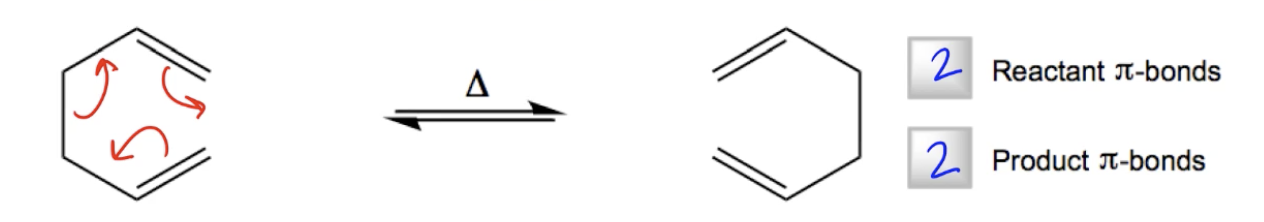2

#### concept

Nomenclature of Sigmatropic Shifts3m
Play a video:

Naming Summary:

• Always described as [x,y]-sigmatropic shifts
1. σ–bond broken = Atom 1
2. σ–bond created = Atoms [x,y]
3
Problem

Provide the correct names and mechanisms for the following sigmatropic shifts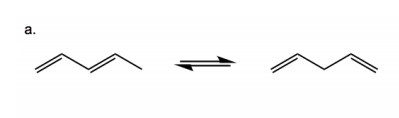4
Problem

Provide the correct names and mechanisms for the following sigmatropic shifts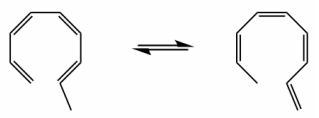5
Problem

Provide the correct names and mechanisms for the following sigmatropic shifts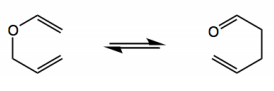6
Problem

Provide the correct names and mechanisms for the following sigmatropic shifts